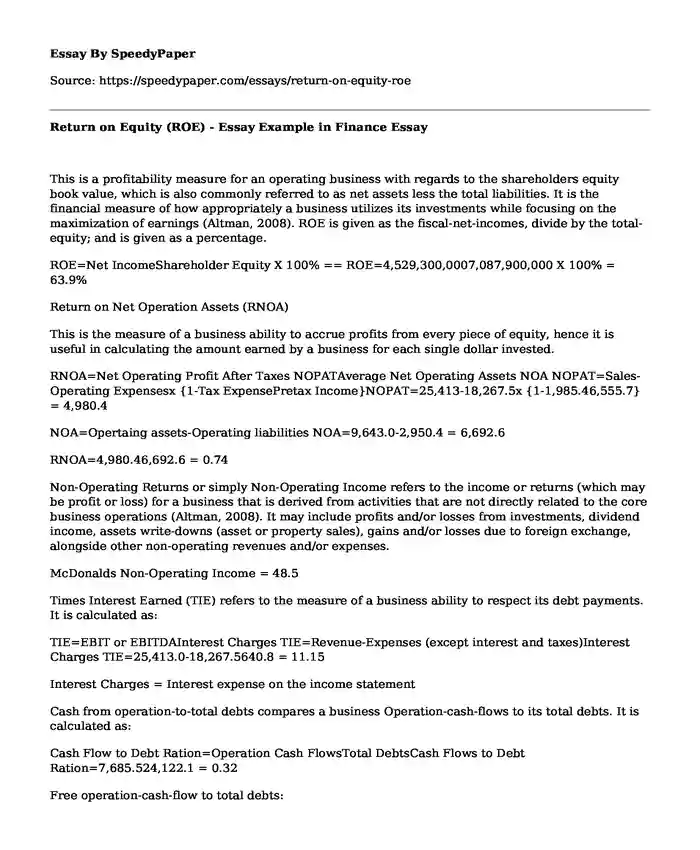# Return on Equity (ROE) - Essay Example in Finance

Published: 2019-11-20 17:42:17Categories: Finance Equity Pages: 2 Wordcount: 488 words
143 views

This is a profitability measure for an operating business with regards to the shareholders equity book value, which is also commonly referred to as net assets less the total liabilities. It is the financial measure of how appropriately a business utilizes its investments while focusing on the maximization of earnings (Altman, 2008). ROE is given as the fiscal-net-incomes, divide by the total-equity; and is given as a percentage.

Is your time best spent reading someone else’s essay? Get a 100% original essay FROM A CERTIFIED WRITER!

ROE=Net IncomeShareholder Equity X 100% == ROE=4,529,300,0007,087,900,000 X 100% = 63.9%

Return on Net Operation Assets (RNOA)

This is the measure of a business ability to accrue profits from every piece of equity, hence it is useful in calculating the amount earned by a business for each single dollar invested.

RNOA=Net Operating Profit After Taxes NOPATAverage Net Operating Assets NOA NOPAT=Sales-Operating Expensesx {1-Tax ExpensePretax Income}NOPAT=25,413-18,267.5x {1-1,985.46,555.7} = 4,980.4

NOA=Opertaing assets-Operating liabilities NOA=9,643.0-2,950.4 = 6,692.6

RNOA=4,980.46,692.6 = 0.74

Non-Operating Returns or simply Non-Operating Income refers to the income or returns (which may be profit or loss) for a business that is derived from activities that are not directly related to the core business operations (Altman, 2008). It may include profits and/or losses from investments, dividend income, assets write-downs (asset or property sales), gains and/or losses due to foreign exchange, alongside other non-operating revenues and/or expenses.

McDonalds Non-Operating Income = 48.5

Times Interest Earned (TIE) refers to the measure of a business ability to respect its debt payments. It is calculated as:

TIE=EBIT or EBITDAInterest Charges TIE=Revenue-Expenses (except interest and taxes)Interest Charges TIE=25,413.0-18,267.5640.8 = 11.15

Interest Charges = Interest expense on the income statement

Cash from operation-to-total debts compares a business Operation-cash-flows to its total debts. It is calculated as:

Cash Flow to Debt Ration=Operation Cash FlowsTotal DebtsCash Flows to Debt Ration=7,685.524,122.1 = 0.32

Free operation-cash-flow to total debts:

Free Cash Flows to Debts Ration=Operation Cash Flow-Capital Expenditure24,122.1Free Cash Flow to Debt Ration=7,685.5-1,813.924,122.1 = 0.24

Z-score: Is the number of SD (standard deviations) for which an observation is above the mean.

It can be obtained as follows:

Z-score (Standard Score) = (1.0E + 0.6D + 3.3C + 1.4B + 1.2A); whereby E = SalesTotal assets; D = Market-value of equityTotal liability; C = Earnings including interest and tax Total-assets; B = Retained earning Total assets; A = Working-capital Total-assets.

A= 1,813.9/37,938.7 = 0.05

B = 44,594.5/37,938.7 = 1.18

C = 7,145.5/37,938.7 = 0.19

D = 7,087.9/30,850.5 = 0.23

E = 16,488.3/37,938.7 = 0.43

Therefore, Z-score = 1.2(0.05) + 1.4(1.18) + 3.3(0.19) + 0.6(0.23) + 1(0.43)

= 0.06 + 1.65 + 0.63 + 0.14 + 0.43

= 2.91

Statement of Income for the fiscal year ended 31, Dec., 2015.

Balance Sheet for the fiscal year-ended 31, Dec., 2015.

Statement of Cash Flow for the fiscal year ended 31, Dec., 2015.

Shareholders Statement of Equity for the fiscal year ended 31, Dec., 2015.

References

Altman, E. I. (2008). Financial ratios, discriminant analysis and the prediction of corporate bankruptcy. The journal of finance, 23(4), 589-609.

Return on Equity (ROE) - Essay Example in Finance. (2019, Nov 20). Retrieved from https://speedypaper.com/essays/return-on-equity-roe

Request Removal

If you are the original author of this essay and no longer wish to have it published on the SpeedyPaper website, please click below to request its removal:

Liked this essay sample but need an original one?

Hire a professional with VAST experience!